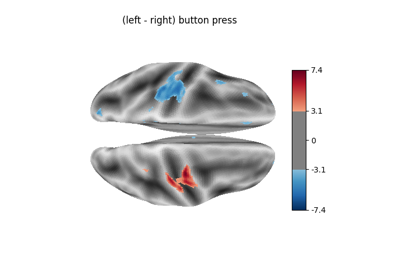Note

This page is a reference documentation. It only explains the function signature, and not how to use it. Please refer to the user guide for the big picture.

# nilearn.glm.compute_contrast#

nilearn.glm.compute_contrast(labels, regression_result, con_val, contrast_type=None)[source]#

Compute the specified contrast given an estimated glm.

Parameters:
labelsarray of shape (n_voxels,)

A map of values on voxels used to identify the corresponding model

regression_resultdict

With keys corresponding to the different labels values are RegressionResults instances corresponding to the voxels.

con_valnumpy.ndarray of shape (p) or (q, p)

Where q = number of contrast vectors and p = number of regressors.

contrast_type{None, ‘t’, ‘F’}, optional

Type of the contrast. If None, then defaults to ‘t’ for 1D con_val and ‘F’ for 2D con_val

Returns:
conContrast instance,

Yields the statistics of the contrast (effects, variance, p-values).

## Examples using `nilearn.glm.compute_contrast`#Example of surface-based first-level analysis

Example of surface-based first-level analysisSurface-based dataset first and second level analysis of a dataset

Surface-based dataset first and second level analysis of a dataset